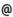## "Babes-Bolyai" University of Cluj-Napoca Faculty of Mathematics and Computer Science

 Computational geometry
 Code Semes-ter Hours: C+S+L Credits Type Section MG263 1 2+2+0 9 compulsory Matematică Computaţională - în limba maghiară
 Teaching Staff in Charge
 Prof. VARGA Csaba Gyorgy, Ph.D., csvargacs.ubbcluj.ro
 Aims The main purpose of the course is the introduction in computational geometry, an important subject for many topics in present applied mathematics and computer science. The seminars gives some impletations by examples, exercices and problems for the results given in the course
 Content I. Basics. 1. Basical algorytms. 2. Geometrical conditions. 3. Calculus methods. II. Intersections. 1. Plane aplications. Intersection of convexe poligons. Intersection of segments. Intersection of half planes. 2. Aplications in space. Intersection of convex poligons. Intersection of half spaces. III. Convex hulls. 1. Construction of convex hulls in plane. 2. Convex hulls in dimensions bigger than two. 3. Aplications in statistics. IV. Problems of nearest points. 1. Problem of the nearest pairs. 2. Voronoi Diagrams. 3. Delaunay triangulation. 4. Generalized Voronoi Diagrams V. Visibility graphs. 1. Shortest paths. 2. Computing the visibility Graph
 References 1. F.P. PREPARATA-M.I. SHAMOS ,Computational Geometry, Springer, 1985. 2. J. O'ROURKE , Computational, Geometry in C, Cambridge, 1993. 3. M. DE BERG , Computational Geometry, Springer, 1997. 4. BOISSONAT, J.-D.- YVINEC, M, Algorithmic Geometry, Cambridge, 1998. 5. CHEN JIANER, Computational Geometry: Methods and Applications, Texas A & M University, 1996. 6. EDELSBRUNNER H., Algorthims in Combinatorial Geometry, Springer-Verlag, Berlin, 1987.
 Assessment 30% from the final mark is the activity during one semester 70% from the final mark is the mark from a written test.